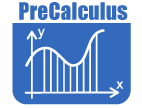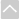# Math - PreCalculusReview Activities and Games

These resources are intended for review and practice after initial instruction of the standards.

Strand: MATHEMATICAL PRACTICES (MP)Strand: NUMBER AND QUANTITY - Vector and Matrix Quantities (N.VM)
Represent and model with vector quantities (Standards 1–3).
Perform operations on vectors (Standards 4–5).
Perform operations on matrices and use matrices in applications (Standards 6–13).Strand: NUMBER AND QUANTITY - Complex Number Systems (N.CN)
Perform arithmetic operations with complex numbers (Standard 3)
Represent complex numbers and their operations on the complex plane (Standards 4–6).
Use complex numbers in polynomial identities and equations (Standard 10).Strand: ALGEBRA: Reasoning With Equations and Inequalities (A.REI)
Solve systems of equations (Standards 8–9).Strand: FUNCTIONS - Interpreting Functions (F.IF)
Analyze functions using different representations (Standard 7, 10–11).Strand: FUNCTIONS - Building Functions (F.BF)
Build a function that models a relationship between two quantities (Standard 1)
Build new functions from existing functions (Standard 4–5).Strand: FUNCTIONS - Trigonometric Functions (F.TF)
Extend the domain of trigonometric functions using the unit circle (Standard 4)
Model periodic phenomena with trigonometric functions (Standard 6–7).
Prove and apply trigonometric identities (Standard 9).Strand: GEOMETRY - Geometric Measurement and Dimension (G.GMD)
Explain volume formulas and use them to solve problems (Standard 2).Strand: GEOMETRY - Expressing Geometric Properties With Equations (G.GPE)
Translate between the geometric description and the equation for a conic section (Standards 2–3).Strand: STATISTICS - Conditional Probability and the Rules of Probability (S.CP)
Understand independence and conditional probability and use them to interpret data (Standards 2–3).
Use the rules of probability to compute probabilities of compound events in a uniform probability model (Standards 7–9).The Online Core Resource pages are a collaborative project between the Utah State Board of Education and the Utah Education Network. If you would like to recommend a high quality resource, contact Trish French (Elementary) or Lindsey Henderson (Secondary). If you find inaccuracies or broken links contact resources@uen.org.